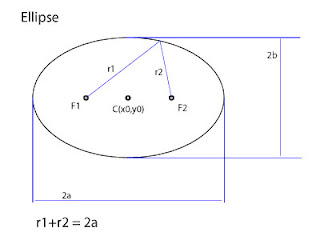First let's start with something we already done here and extend our knowledge. Take a look at previous posts about circular movement in ActionScript 3.0:

How to orbit in AS
Making orbit trails in Flash
Using drawing API for orbit like shapes

### Elliptic movement in AS3Ellipse has center point C(x0,y0) and two fixed points F1 and F2. Distances from F1 and F2 are r1 and r2. You can think of circular movement as special case of ellipse movement where values a and b are equal (a=b).

Thus, based on previous examples we can write code for finding position of point moving along elliptic curve.

var posX:Number = planet.x + Math.cos(radians) * a;
var posY:Number = planet.y + Math.sin(radians) * b;

Instead of single radius parameter in orbit function, ellipse function has two parameters a and b.

``function ellipse(planet:MovieClip, sat:MovieClip, a:Number, b:Number, speed:Number):void{    var currentDegrees:Number = 0;    this.addEventListener(Event.ENTER_FRAME, doEveryFrame);    function doEveryFrame(event:Event):void    {        currentDegrees += speed;        var radians:Number = getRadians(currentDegrees);        var posX:Number = planet.x + Math.cos(radians) * a;        var posY:Number = planet.y + Math.sin(radians) * b;        sat.x = posX;        sat.y = posY;    }        function getRadians(degrees:Number):Number    {        return degrees * Math.PI / 180;    }    }``

That's all. You can replace orbit function with ellipse one in previous trails examples and it will work perfectly.

*_*

1.Thanks for the code explanation

visit my blog too..

designscripting.com

2.Hello again,

I've been doing something similar to this recently and thought I'd contribute a bit

Ellipse based spaceship orbit

My coding's a bit messy in the project but here's the bulk of my ellipse class (it has rotation functionality!)

script:

var h=100;
var w=200;
var ang=Math.PI/2;
var steps=50;
function loop(e:Event)
{
graphics.clear();
graphics.lineStyle(1,0xFFFFFF);
for (var i=0; i<=steps; i++)
{
var stepang=(2*Math.PI*i/steps);
var x1=w*Math.cos(stepang);
var y1=h*Math.sin(stepang);
var x2=x1*Math.cos(ang)-y1*Math.sin(ang);
var y2=x1*Math.sin(ang)+y1*Math.cos(ang);
if (i==0) graphics.moveTo(x2,y2);
graphics.lineTo(x2,y2);
}
}

Make a new MovieClip, paste that in, enjoy the result ;)

-Ant

3.Hi Ant,

seems like we have similar interests :)

good example there on your blog, I can already see some types of games can use your elliptical movement code.

can't wait for your video tutorial
have a great holiday ;)

Flanture

Thanks for sharing your thoughts !